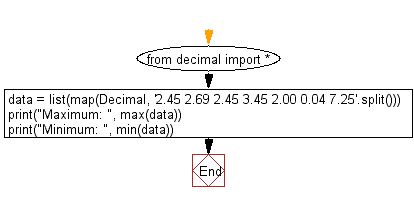﻿ Python Math: Find the maximum and minimum numbers from the specified decimal numbers - w3resource# Python Math: Find the Maximum and minimum numbers from the specified decimal numbers

## Python Math: Exercise-36 with Solution

Write a Python program to find the maximum and minimum numbers from the specified decimal numbers.

Sample Solution:-

Python Code:

``````from decimal import *
data = list(map(Decimal, '2.45 2.69 2.45 3.45 2.00 0.04 7.25'.split()))
print("Maximum: ", max(data))
print("Minimum: ", min(data))
```
```

Sample Output:

```Maximum:  7.25
Minimum:  0.04
```

Pictorial Presentation:Flowchart:Python Code Editor:

Have another way to solve this solution? Contribute your code (and comments) through Disqus.

What is the difficulty level of this exercise?

Test your Programming skills with w3resource's quiz.

﻿

## Python: Tips of the Day

Debug With the Print() Function:

```>>> for i in range(5):
...     print(i, end=', ' if i < 4 else '\n')
...
0, 1, 2, 3, 4
>>> for i in range(5):
...     print(f'{i} & {i*i}', end=', ' if i < 4 else '\n')
...
0 & 0, 1 & 1, 2 & 4, 3 & 9, 4 & 16
```